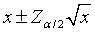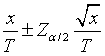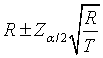# Confidence interval for a rate

## 2007-10-10

### Dear Professor Mean,

How do you calculate a confidence interval for a rate?

First, you need to carefully define what a rate is.

A rate, as I understand the word, is a count divided by a value in a different unit of measure, such as time or area. So the number of people per square mile or the number of murders per year are rates. A rate could also involve dividing a count of one quantity by a count of another quantity. So the number of cars per person is also a rate.

A rate is different from a proportion where the numerator and denominator are both counts and where the numerator is a subset of the denominator. So the number of children born with birth defects divided by the number of live births is a proportion, not a rate. Lots of people use the term rate to mean either a rate or a proportion. For example, a survey return rate (the number of surveys returned divided by the number sent out) is actually a survey return proportion.

The simplest way to get a confidence interval for a single rate is to treat the denominator as a fixed quantity and treat the numerator as a Poisson random variable. Since the standard deviation of a Poisson random variable is simply the square root of the expected value, you can use the simple approximate formulaas a confidence interval for a Poisson count variable equal to x. Then simply divide the limits of the confidence interval by the same quantity that you divided the count by the same denominator. This produces the following formulaIf you let R=x/T, then this formula simplifies to### Example

In a study of AIDS patients, six cases of tuberculosis were noted in 162.2 patient years of observation (PYO). This leads to a rate of 3.7 per 100 PO. The 95% confidence interval for a Poisson count of 6 is 6 +/- 1.96*sqrt(6) = 1.2 to 10.8. The 95% confidence interval for the rate of 3.7 requires us to divide the confidence limits above by 162.2/100. This gives you limits of 0.7 and 6.7. Obviously these intervals are quite wide, as they should be since they are based on only 6 cases. The limits for the Poisson distribution are only approximate, of course, and a weak approximation when the count is very low. Nevertheless, this interval gives you a quick indication of the amount of uncertainty associated with this rate.

Source: Antiretroviral therapy at a district hospital in Ethiopia prevents death and tuberculosis in a cohort of HIV patients**. Jerene, D., A. Naess, et al. AIDS Res Ther 2006; 3: 10. Available in html format or pdf format.

I’ve written a simple Excel spreadsheet to calculate a confidence interval for a single rate.

If the statistic in question is a proportion rather than a rate, then you should use the formula for a confidence interval for a single proportion.though if the proportion is small and the sample size is large, the confidence interval for a rate can be used as simple and fairly accurate approximation.

You can find an earlier version of this page on my old website.